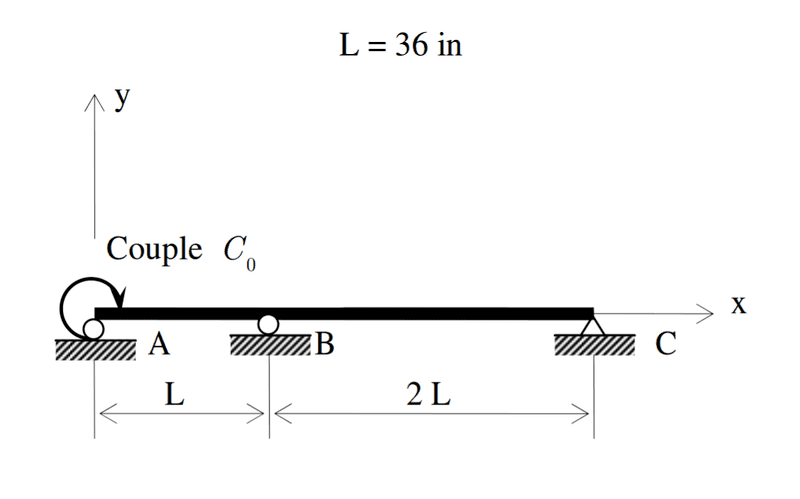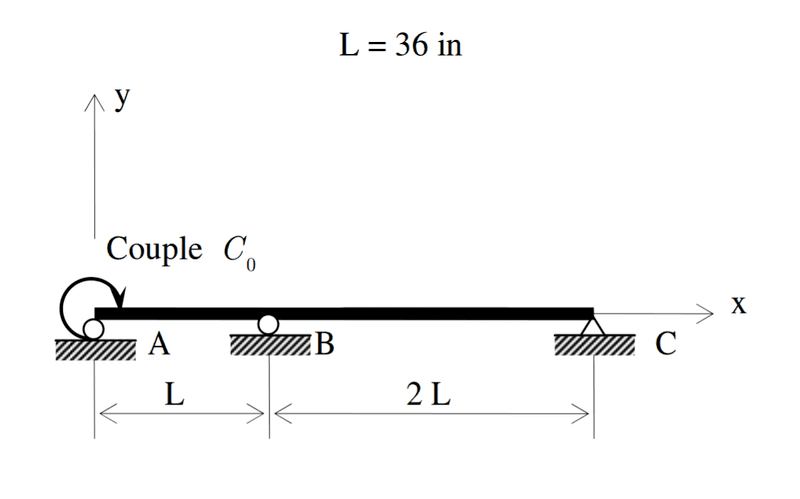# Statics problem(simple beam/three supports/one moment)

• Halest0rm3
In summary, the conversation discusses the problem of solving for the reaction forces Ay, By, and Cy, with the presence of a clockwise couple at point A. The equations of static equilibrium are used to try and solve for the reactions, but due to the beam being statically indeterminate, a third equation is needed to solve for the unknown reactions. This can be found by using a different method that does not involve the sum of forces or moments.

#### Halest0rm3## Homework Statement

I have to solve for Ay, By, and Cy reaction forces. The constant C is a couple acting at A in a clockwise direction

## Homework Equations

Sum of all forces = 0
Sum of Moments about any point = 0

## The Attempt at a Solution

I solved for all four reaction equations(one force sum and three moment equations at a,b, and c) These are the four equations I calculated:

Ay + By + Cy = 0
36(By) + 3(36)(Cy) = C
2(36)(Cy) - 36(Ay) = C
-3(36)(Ay) -2(36)(By) = C

But no matter how I try to solve the system of equations I always get 0 = 0 or a singular matrix. I don't know if the problem is that I messed up with one of the moment equations or if there is something else I need to do. Any help would be very much appreciated, thanks!

Halest0rm3 said:## Homework Statement

I have to solve for Ay, By, and Cy reaction forces. The constant C is a couple acting at A in a clockwise direction

## Homework Equations

Sum of all forces = 0
Sum of Moments about any point = 0

## The Attempt at a Solution

I solved for all four reaction equations(one force sum and three moment equations at a,b, and c) These are the four equations I calculated:

Ay + By + Cy = 0
36(By) + 3(36)(Cy) = C
2(36)(Cy) - 36(Ay) = C
-3(36)(Ay) -2(36)(By) = C

But no matter how I try to solve the system of equations I always get 0 = 0 or a singular matrix. I don't know if the problem is that I messed up with one of the moment equations or if there is something else I need to do. Any help would be very much appreciated, thanks!
This beam is statically indeterminate. You can write only two equations of static equilibrium, but you have 3 unknown reactions to solve for.

You'll have to find a third equation to use which doesn't involve the sum of the forces or the sum of the moments.

https://en.wikipedia.org/wiki/Statically_indeterminate